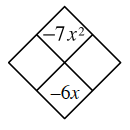### Home > INT2 > Chapter 4 > Lesson 4.2.4 > Problem4-99

4-99.

Copy and complete each Diamond Problem below. The pattern used in the Diamond Problems is shown at right.

1.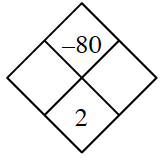Which two integers have a product of $−80$ and a sum of $2$?

1.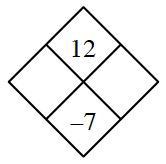1.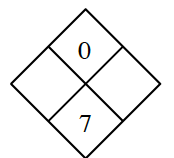1.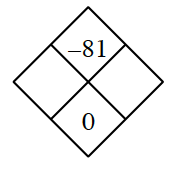Think of opposites that are factors of $−81$.

1.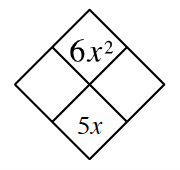Imagine the $x$'s are not there.
Which two numbers multiply to $6$ and add to $5$?

1.